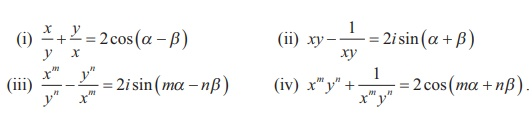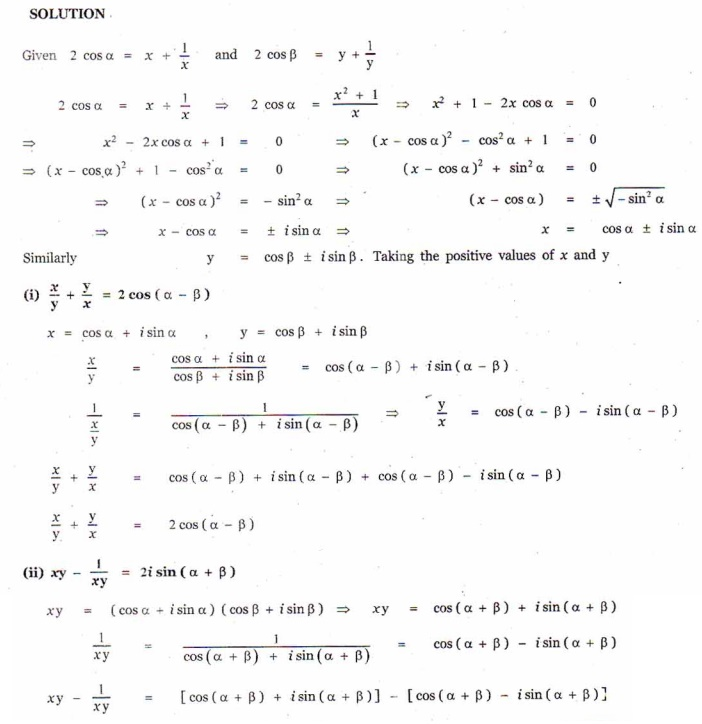Home | | Maths 12th Std | Exercise 2.8: de MoivreŌĆÖs Theorem and its Applications

# Exercise 2.8: de MoivreŌĆÖs Theorem and its Applications

Maths Book back answers and solution for Exercise questions - Mathematics : Complex Numbers: de MoivreŌĆÖs Theorem and its Applications : Exercise problems with Questions, Answers, Solution, Explanation

EXERCISE 2.8

1. If Žē ŌēĀ 1 is a cube root of unity, show that2. Show that3. Find the value of4. If 2cos╬▒ = x + [1/x] and 2cos ╬▓ = y + [1/y] , show that5. Solve the equation z3 + 27 = 0 .6. If Žē ŌēĀ 1 is a cube root of unity, show that the roots of the equation ( z -1)3 + 8 = 0 are -1, 1- 2Žē, 1- 2Žē2 .7. Find the value of8. If Žē ŌēĀ 1 is a cube root of unity, show that

(i) (1- Žē + Žē2)6 + (1+ Žē - Žē2 )6 = 128.

(ii) (1+ Žē )(1+ Žē2)(1+ Žē4 )(1+ Žē8 )... ...(1+ Žē 2.pow(11) ) = 1.9. If z = 2 - 2i , find the rotation of z by ╬Ė radians in the counter clockwise direction about the origin when

(i) ╬Ė = ŽĆ/3

(ii) ╬Ė = 2ŽĆ/3

(iii) ╬Ė = 3ŽĆ ./210. Prove that the values of 4ŌłÜ-1 are ┬▒ 1/ŌłÜ2 (1┬▒i).